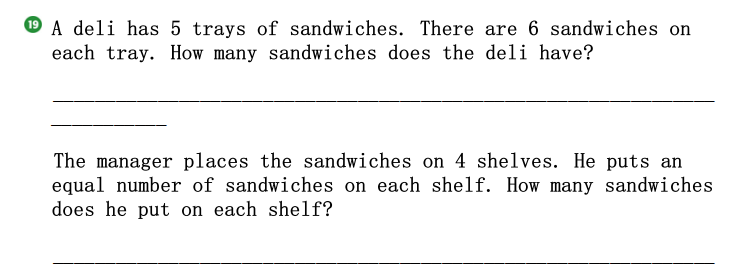### ¿Todavía tienes preguntas de matemáticas?

Pregunte a nuestros tutores expertos
Algebra
PreguntaA deli has $$5$$ trays of sandwiches. There are $$6$$ sandwiches on each tray. How many sandwiches does the deli have?

The manager places the sandwiches on $$4$$ shelves. He puts an equal number of sandwiches on each shelf. How many sandwiches does he put on each shelf?